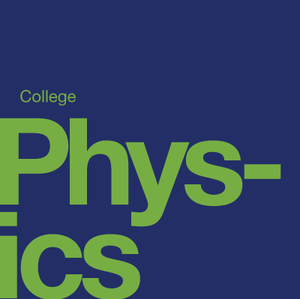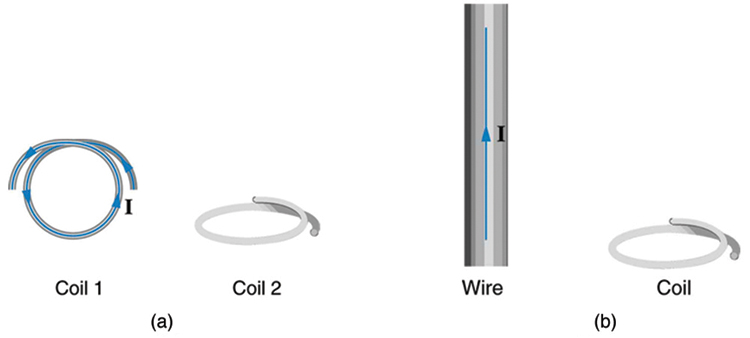# Electromagnetic Induction and Technologies

Source: OpenStax

Student Price: FREE

This question pack examines emf and magnetic flux, Faraday's Law, motional emf, eddy currents, electric generators, transformers and more.

PE 23.1

What is the value of the magnetic flux at coil 2 in Figure due to coil 1? Figure note: (a) The planes of the two coils are perpendicular. (b) The wire is perpendicular to the plane of the coil.PE 23.2

What is the value of the magnetic flux through the coil in Figure (b) due to the wire?

Figure note: (a) The planes of the two coils are perpendicular. (b) The wire is perpendicular to the plane of the coil.PE 23.8

Suppose a 50-turn coil lies in the plane of the page in a uniform magnetic field that is directed into the page. The coil originally has an area of 0.250 m$^2$. It is stretched to have no area in 0.100 s. What is the direction and magnitude of the induced emf if the uniform magnetic field has a strength of 1.50 T? (in VCW)

PE 23.11

An emf is induced by rotating a 1000-turn, 20.0 cm diameter coil in the Earth’s 5.00×10$^{−5}$ T magnetic field. What average emf is induced, given the plane of the coil is originally perpendicular to the Earth’s field and is rotated to be parallel to the field in 10.0 ms? (in V)

PE 23.12

A 0.250 m radius, 500-turn coil is rotated one-fourth of a revolution in 4.17 ms, originally having its plane perpendicular to a uniform magnetic field. (This is 60 rev/s.) Find the magnetic field strength needed to induce an average emf of 10,000 V. (in T)

PE 23.19

At what speed must the sliding rod in Figure 23.11 move to produce an emf of 1.00 V in a 1.50 T field, given the rod’s length is 30.0 cm? (in m/s)

PE 23.20

The 12.0 cm long rod in Figure 23.11 moves at 4.00 m/s. What is the strength of the magnetic field if a 95.0 V emf is induced? (in T)

PE 23.22

In the August 1992 space shuttle flight, only 250 m of the conducting tether considered in Example 23.2 could be let out. A 40.0 V motional emf was generated in the Earth’s 5.00 x 10$^{-5}$ T field, while moving at 7.80 x 10$^3$ m/s . What was the angle between the shuttle’s velocity and the Earth’s field, assuming the conductor was perpendicular to the field? (in $^0$)

PE 23.28

Calculate the peak voltage of a generator that rotates its 200-turn, 0.100 m diameter coil at 3600 rpm in a 0.800 T field. (in V)

PE 23.29

At what angular velocity in rpm will the peak voltage of a generator be 480 V, if its 500-turn, 8.00 cm diameter coil rotates in a 0.250 T field? (in rpm)

PE 23.30

What is the peak emf generated by rotating a 1000-turn, 20.0 cm diameter coil in the Earth’s 5.00×10$^{−5}$ T magnetic field, given the plane of the coil is originally perpendicular to the Earth’s field and is rotated to be parallel to the field in 10.0 ms? (in V)

PE 23.31

What is the peak emf generated by a 0.250 m radius, 500-turn coil is rotated one-fourth of a revolution in 4.17 ms, originally having its plane perpendicular to a uniform magnetic field. (This is 60 rev/s.) (in kV)

PE 23.41

What is the back emf of a 120 V motor that draws 8.00 A at its normal speed and 20.0 A when first starting? (in V)

PE 23.42

The motor in a toy car operates on 6.00 V, developing a 4.50 V back emf at normal speed. If it draws 3.00 A at normal speed, what current does it draw when starting? (in A)

PE 23.43

The motor in a toy car is powered by four batteries in series, which produce a total emf of 6.00 V. The motor draws 3.00 A and develops a 4.50 V back emf at normal speed. Each battery has a 0.100 Ω internal resistance. What is the resistance of the motor (in ohms)?

PE 23.55

Two coils are placed close together in a physics lab to demonstrate Faraday’s law of induction. A current of 5.00 A in one is switched off in 1.00 ms, inducing a 9.00 V emf in the other. What is their mutual inductance (in mH)?

PE 23.56

If two coils placed next to one another have a mutual inductance of 5.00 mH, what voltage is induced in one when the 2.00 A current in the other is switched off in 30.0 ms? (in V)

PE 23.57

The 4.00 A current through a 7.50 mH inductor is switched off in 8.33 ms. What is the emf induced opposing this? (in V)

PE 23.58

A device is turned on and 3.00 A flows through it 0.100 ms later. What is the self-inductance of the device if an induced 150 V emf opposes this? (in mH)

PE 23.65

When the 20.0 A current through an inductor is turned off in 1.50 ms, an 800 V emf is induced, opposing the change. What is the value of the self-inductance (in mH)?

PE 23.69

If you want a characteristic RL time constant of 1.00 s, and you have a 500 Ω resistor, what value of self-inductance is needed (in H)?

PE 23.71

A large superconducting magnet, used for magnetic resonance imaging, has a 50.0 H inductance. If you want current through it to be adjustable with a 1.00 s characteristic time constant, what is the minimum resistance of system (in ohms)?

PE 23.79

At what frequency will a 30.0 mH inductor have a reactance of 100 Ω (in Hz)?

PE 23.80

What value of inductance should be used if a 20.0 kΩ reactance is needed at a frequency of 500 Hz? (in H)

PE 23.81

What capacitance should be used to produce a 2.00 MΩ reactance at 60.0 Hz (in nF)?

PE 23.82

At what frequency will an 80.0 mF capacitor have a reactance of 0.250 Ω? (in Hz)

PE 23.85

A 20.0 kHz, 16.0 V source connected to an inductor produces a 2.00 A current. What is the inductance (in µH)?

PE 23.86

A 20.0 Hz, 16.0 V source produces a 2.00 mA current when connected to a capacitor. What is the capacitance? (in µF)

PE 23.95

What is the resonant frequency of a 0.500 mH inductor connected to a 40.0 μF capacitor? (in kHz)

PE 23.97

Suppose you have a supply of inductors ranging from 1.00 nH to 10.0 H, and capacitors ranging from 1.00 pF to 0.100 F. What is the range of resonant frequencies that can be achieved from combinations of a single inductor and a single capacitor? (in GHz)

PE 23.98

What capacitance do you need to produce a resonant frequency of 1.00 GHz, when using an 8.00 nH inductor (in pF)?

PE 23.99

What inductance do you need to produce a resonant frequency of 60.0 Hz, when using a 2.00 μF capacitor? (in H)

CC BY - OpenStax